# Video encoding in simple terms

Video encoding in simple terms

### CHAPTER 1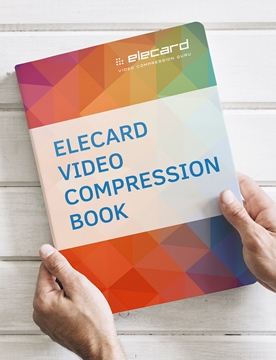### Basic information about video compression technology: brief history, stages of video encoding, main algorithms of AVC/H.264 and HEVC/H.265 standards.

Nowadays, it is difficult to imagine a field of human activity, in which, in one way or another, digital video has not entered. We watch it on TV, mobile devices, and stationary computers; we record it with digital cameras ourselves, or we encounter it on the roads (unpleasant, but true), in stores, hospitals, schools and universities, and in industrial enterprises of various profiles. As a consequence, words and terms that are directly related to the digital representation of video information are becoming more firmly and widely embedded in our lives. From time to time, questions arise in this area. What are the differences between various devices or programs that we use to encode/ decode digital video data, and what do they do? Which of these devices/ programs are better or worse, and in which aspects? What do all these endless MPEG-2, H.264 / AVC, VP9, H.265 / HEVC, etc. mean? Let's try to understand.

### A very brief historical reference

The first generally accepted video compression standard MPEG-2 was finally adopted in 1996, after which a rapid development of digital satellite television began. The next standard was MPEG-4 part 10 (H.264 / AVC), which provides twice the degree of video data compression. It was adopted in 2003, which led to the development of DVB-T/ C systems, Internet TV and the emergence of a variety of video sharing and video communication services. From 2010 to 2013, the Joint Collaborative Team on Video Coding (JCT-VC) was intensively working to create the next video compression standard, which was called High Efficient Video Coding (HEVC) by the developers; it ensured the following twofold increase in the compression ratio of digital video data. This standard was approved in 2013. That same year, the VP9 standard, developed by Google, was adopted, which was supposed to not yield to HEVC in its degree of video data compression.

### Basic stages of video encoding

There are a few simple ideas at the core of algorithms for video data compression. If we take some part of an image (in the MPEG-2 and AVC standards this part is called a macroblock), then there is a big possibility that, near this segment  in this frame or in neighboring frames, there will be a segment containing a similar image, which differs little in pixel intensity values. Thus, to transmit information about the image in the current segment, it is enough to only transfer its difference from the previously encoded similar segment. The process of finding similar segments among previously encoded images is called Prediction. A set of difference values that determine the difference between the current segment and the found prediction is called the Residual. Here we can distinguish two main types of prediction. In the first one, the Prediction values represent a set of linear combinations of pixels adjacent to the current image segment on the left and on the top. This type of prediction is called Intra Prediction. In the second one, linear combinations of pixels of similar image segments from previously encoded frames are used as prediction (these frames are called Reference). This type of prediction is called Inter Prediction. To restore the image of the current segment, encoded with Inter prediction, when decoding, it is necessary to have information about not only the Residual, but also the frame number, where a similar segment is located, and the coordinates of this segment.

Residual values obtained during prediction obviously contain, on average, less information than the original image and, therefore, require a fewer quantity of bits for image transmission. To further increase the degree of compression of video data in video coding systems, some spectral transformation is used. Typically, this is Fourier cosine transform. Such transformation allows us to select the fundamental harmonics in two-dimensional Residual signal. Such a selection is made at the next stage of coding - quantization. The sequence of quantized spectral coefficients contains a small number of main, large values. The remaining values are very likely to be zero. As a result, the amount of information contained in quantized spectral coefficients is significantly (dozens of times) lower than in the original image.

In the next stage of coding, the obtained set of quantized spectral coefficients, accompanied by the information necessary for performing prediction when decoding, is subjected to entropy coding. The bottom line here is to align the most common values of the encoded stream with the shortest codeword (containing the smallest number of bits). The best compression ratio (close to theoretically achievable) at this stage is provided by arithmetic coding algorithms, which are mainly used in modern video compression systems.

From the above, the main factors affecting the effectiveness of a particular video compression system become apparent. First of all, these are, of course, the factors that determine the effectiveness of the Intra and Inter Predictions. The second set of factors is related to the orthogonal transformation and quantization, which selects the fundamental harmonics in the Residual signal. The third one is determined by the volume and compactness of the representation of additional information accompanying Residual and necessary for making predictions, that is, calculating Prediction, in the decoder. Finally, the fourth set has the factors that determine the effectiveness of the final stage- entropy coding.

Let's illustrate some possible options (far from all) of the implementation of the coding stages listed above, on the example of H.264 / AVC and HEVC.

### AVC Standard

In the AVC standard, the basic structural unit of the image is a macroblock - a square area of 16x16 pixels (Figure 1). When searching for the best possible prediction, the encoder can select one of several options of partitioning each macroblock. With Intra-prediction, there are three options: perform a prediction for the entire block as a whole, break the macroblock into four square blocks of 8x8 size, or into 16 blocks with a size of 4x4 pixels, and perform a prediction for each such block independently. The number of possible options of macroblock partitioning under Inter-prediction is much richer (Figure 1), which provides adaptation of the size and position of the predicted blocks to the position and shape of the object boundaries moving in the video frame.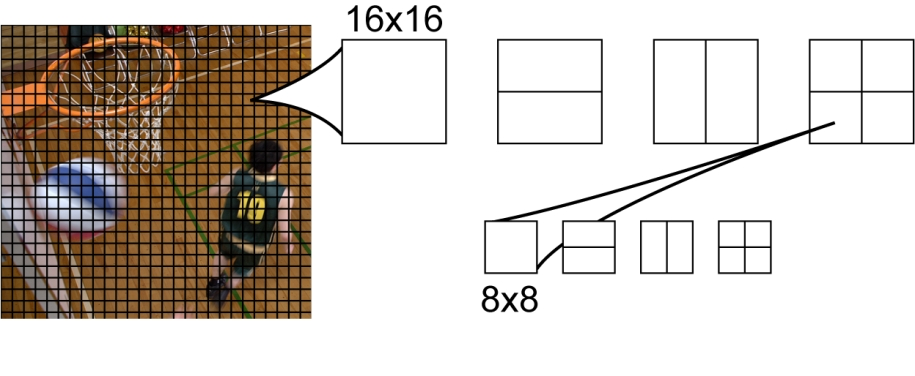Fig 1. Macroblocks in AVC and possible partitioning when using Inter-Prediction.

In AVC, pixel values from the column to the left of the predicted block and the row of pixels immediately above it are used for Intra prediction (Figure 2). For blocks of sizes 4x4 and 8x8, 9 methods of prediction are used. In a prediction called DC, all calculated pixels have a single value equal to the arithmetic average of the "neighbor pixels" highlighted in Fig. 2 with a bold line. In other modes, "angular" prediction is performed. In this case, the values of the "neighbor pixels" are placed inside the predicted block in the directions indicated in Fig. 2.

In the event that the predicted pixel gets between "neighbor pixels", when moving in a given direction, an interpolated value is used for the prediction. For blocks with a size of 16x16 pixels, 4 methods of prediction are used. One of them is the DC-prediction, which was already reviewed. The other two correspond to the "angular" methods, with the directions of prediction 0 and 1. Finally, the fourth - Plane-prediction : the values of the predicted pixels are determined by the equation of the plane. The angular coefficients of the equation are determined by the values of the "neighboring pixels".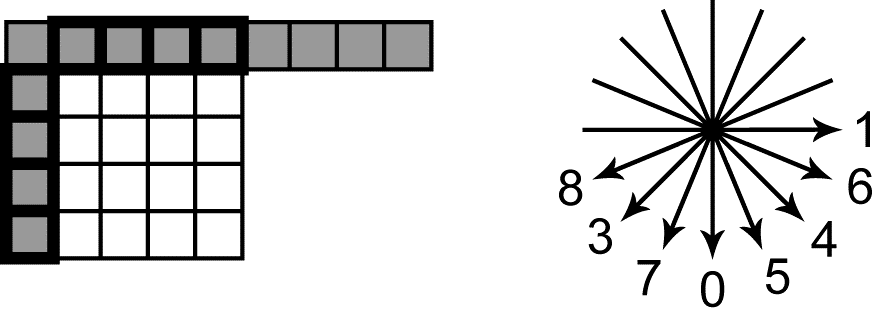Fig 2. “Neighboring pixels” and angular modes of Intra-Prediction in AVC

Inter- Prediction in AVC can be implemented in one of two ways. Each of these options determines the type of macroblock (P or B). As a prediction of pixel values in P-blocks (Predictive-blocks), the values of pixels from the area located on the previously coded (reference) image, are used. Reference images are not deleted from the RAM buffer, containing decoded frames (decoded picture buffer, or DPB), as long as they are needed for Inter-prediction. A reference list is created in the DPB from the indexes of these images.

The encoder signals to the decoder about the number of the reference image in the list and about the offset of the area used for prediction, with respect to the position of predicted block (this displacement is called motion vector). The offset can be determined with an accuracy of ¼ pixel. In case of prediction with non-integer offset, interpolation is performed. Different blocks in one image can be predicted by areas located on different reference images.

In the second option of Inter Prediction, prediction of the B-block pixel values (bi-predictive block), two reference images are used; their indexes are placed in two lists (list0 and list1) in the DPB. The two indexes of reference images in the lists and two offsets, that determine positions of reference areas, are transmitted to the decoder. The B-block pixel values are calculated as a linear combination of pixel values from the reference areas. For non-integer offsets, interpolation of reference image is used.

As already mentioned, after predicting the values of the encoded block and calculating the Residual signal, the next coding step is spectral transformation. In AVC, there are several options for orthogonal transformations of the Residual signal. When Intra-prediction of a whole macroblock with a size of 16x16 is implemented, the residual signal is divided into 4x4 pixel blocks; each of them is subjected to an integer analog of  discrete two-dimensional 4x4 cosine Fourier transform.

The resulting spectral components, corresponding to zero frequency (DC) in each block, are then subjected to additional orthogonal Walsh-Hadamard transform. With Inter-prediction, the Residual signal is divided into blocks of 4x4 pixels or 8x8 pixels. Each block is then subjected to a 4x4 or 8x8 (respectively) two-dimensional discrete cosine Fourier Transform (DCT, from Discrete Cosine Transform).

In the next step, spectral coefficients are subjected to the  quantization procedure. This leads to a decrease in bit capacity of digits representing the spectral sample values, and to a significant increase in the number of samples having zero values. These effects provide compression, i.e. reduce the number and bit capacity of digits representing the encoded image. The reverse side of quantization is the distortion of the encoded image. It is clear that the larger the quantization step, the greater is the compression ratio, but also the distortion is greater.

The final stage of encoding in AVC is entropy coding, implemented by the algorithms of Context Adaptive Binary Arithmetic Coding. This stage provides additional compression of video data without distortion in the encoded image.

### Ten years later. HEVC standard: what's new?

The new H.265/HEVC standard is the development of methods and algorithms for compressing video data embedded in H.264/AVC. Let's briefly review the main differences.

An analog of a macroblock in HEVC is the Coding Unit (CU). Within each block, areas for calculation of Prediction are selected - Prediction Unit (PU). Each CU also specifies the limits within which the areas for calculating the discrete orthogonal transformation from the residual signal are selected. These areas are called the Transform Unit (TU).

The main distinguishing feature of HEVC here is that the split of a video frame into CU is conducted adaptively, so that it is possible to adjust the CU boundaries to the boundaries of objects on the image (Figure 3). Such adaptability allows to achieve an exceptionally high quality of prediction and, as a consequence, a low level of the residual signal.

An undoubted advantage of such an adaptive approach to frame division into blocks is also an extremely compact description of the partition structure. For the entire video sequence, the maximum and minimum possible CU sizes are set (for example, 64x64 is the maximum possible CU, 8x8 is the minimum). The entire frame is covered with the maximum possible CUs, left to right, top-to-bottom.

It is obvious that, for such coverage, transmission of any information is not required. If partition is required within any CU, then this is indicated by a single flag (Split Flag). If this flag is set to 1, then this CU is divided into 4 CUs (with a maximum CU size of 64x64, after partitioning we get 4 CUs of size 32x32 each).

For each of the CUs received, a Split Flag value of 0 or 1 can, in turn, be transmitted. In the latter case, this CU is again divided into 4 CUs of smaller size. The process continues recursively until the Split Flag of all received CUs is equal to 0 or until the minimum possible CU size is reached. Inserted CUs thus form a quad tree (Coding Tree Units, CTU).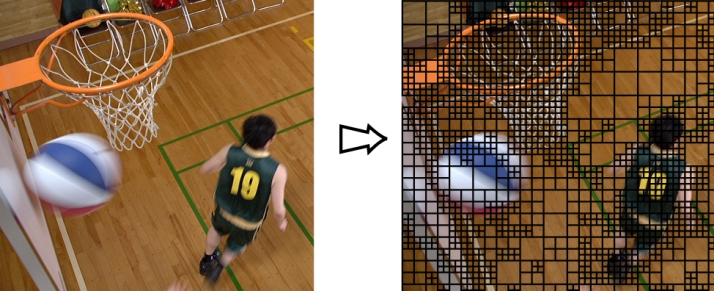Fig.3 Video frame partitioning into CUs is conducted adaptively

As already mentioned, within each CU, areas for calculating prediction- Prediction Units (PU) are selected. With Intra Prediction, the CU area can coincide with the PU (2Nx2N mode) or it can be divided into 4 square PUs of twice smaller size (NxN mode, available only for CU of minimum size). With Inter Prediction, there are eight possible options for partitioning each CU into PUs (Figure 3).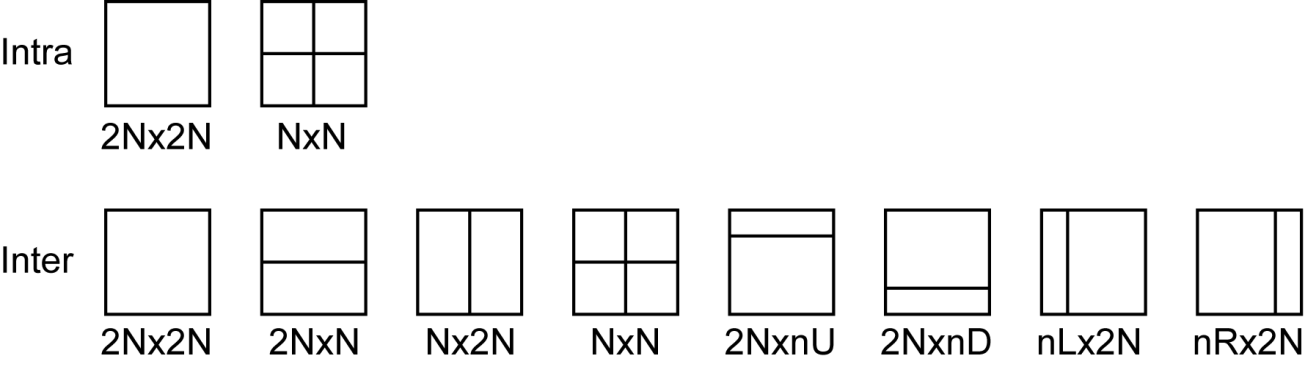Pic. 4. Possible partitioning of the Coding Unit into Prediction Units with the spatial (Intra) and temporary (Inter) CU prediction modes

The idea of spatial prediction in HEVC remained the same as in AVC. Linear combinations of neighboring pixel values, adjacent to the block on the left and above, are used as predicted sample values in the PU block. However, the set of methods for spatial prediction in HEVC has become significantly richer. In addition to Planar (analogue to Plane in AVC) and DC methods, each PU can be predicted by one of the 33 ways of "angular" prediction.  That is, the number of ways, in which the values are calculated by "neighbor”-pixels, is increased by 4 times.

We can point out two main differences of Inter- prediction between HEVC and AVC. Firstly, HEVC uses better interpolation filters (with a longer impulse response) when calculating reference images with non-integer offset. The second difference concerns the way the information about the reference area, required by the decoder for performing the prediction, is presented. In HEVC, a "merge mode" is introduced, where different PUs, with the same offsets of reference areas, are combined. For the entire combined area, information about motion (motion vector) is transmitted in the stream once, which allows a significant reduction in the amount of information transmitted.

In HEVC, the size of the discrete two-dimensional transformation, to which the Residual signal is subjected, is determined by the size of the square area called the Transform Unit (TU). Each CU is the root of the TU quad tree. Thus, the TU of the upper level coincides with the CU. The root TU can be divided into 4 parts of half the size, each of which, in turn, is a TU and can be further divided.

The size of discrete transformation is determined by the TU size of the lower level. In HEVC, transforms for blocks of 4 sizes are defined: 4x4, 8x8, 16x16, and 32x32. These transformations are integer analogs of the discrete two-dimensional Fourier cosine transform of corresponding size. For size 4x4 TU with Intra-prediction, there is also a separate discrete transformation, which is an integer analogue of the discrete sine Fourier transform.

The ideas of the procedure of quantizing spectral coefficients of Residual signal, and also entropy coding in AVC and in HEVC, are practically identical.

Let's note one more point which was not mentioned before. The quality of decoded images and the degree of video data compression are influenced significantly by post-filtering, which decoded images with Inter-prediction undergo before they are placed in the DPB.

In AVC, there is one kind of such filtering - deblocking filter. Application of this filter reduces the block effect resulting from quantization of spectral coefficients after orthogonal transformation of Residual signal.

In HEVC, a similar deblocking filter is used. Besides, an additional non-linear filtering procedure called the Sample Adaptive Offset (SAO) exists. Based on the analysis of pixel value distribution during encoding, a table of corrective offsets, added to the values of a part of CU pixels during decoding, is determined.

In HEVC, the size of the discrete two-dimensional transformation, to which the Residual signal is subjected, is determined by the size of the square area called the Transform Unit (TU). Each CU is the quad-tree of TU’s. Thus, the TU of the upper level coincides with the CU. The root TU can be divided into 4 parts of half the size, each of which, in turn, is a TU and can be further divided.

The size of discrete transformation is determined by the TU size of the lower level. There are four transform block sizes in HEVC: 4x4, 8x8, 16x16, and 32x32. These transforms are discrete two-dimensional Fourier cosine transform of corresponding size. For 4x4 Intra-predicted blocks, could be used another discrete transform - sine Fourier transform.

The quantization of spectral coefficients of residual signal, and entropy coding in AVC and in HEVC, are almost identical.

Let's note one more point which was not mentioned before. The quality of decoded images, hence the degree of video data compression, is influenced significantly by post-filtering, which applied on decoded Inter-predicted images before they are placed in the DPB.

In AVC, there is one kind of such filtering - deblocking filter.  It masking blocking artifacts effect originating from spectral coefficients quantization after orthogonal transformation of residual signal.

In HEVC, a similar deblocking filter is used. Besides, an additional non-linear filtering procedure called the Sample Adaptive Offset (SAO) exists. Sample level correction is based either on local neighborhood or on the intensity level of sample itself. Table of sample level corrections, added to the values of a part of CU pixels during decoding, is determined.

### And what is the result?

Figures 4-7 show the results of encoding of several high-resolution (HD) video sequences by two encoders. One of the encoders compresses the video data in the H.265/HEVC standard (marked as HM on all the graphs), and the second one is in the H.264/AVC standard.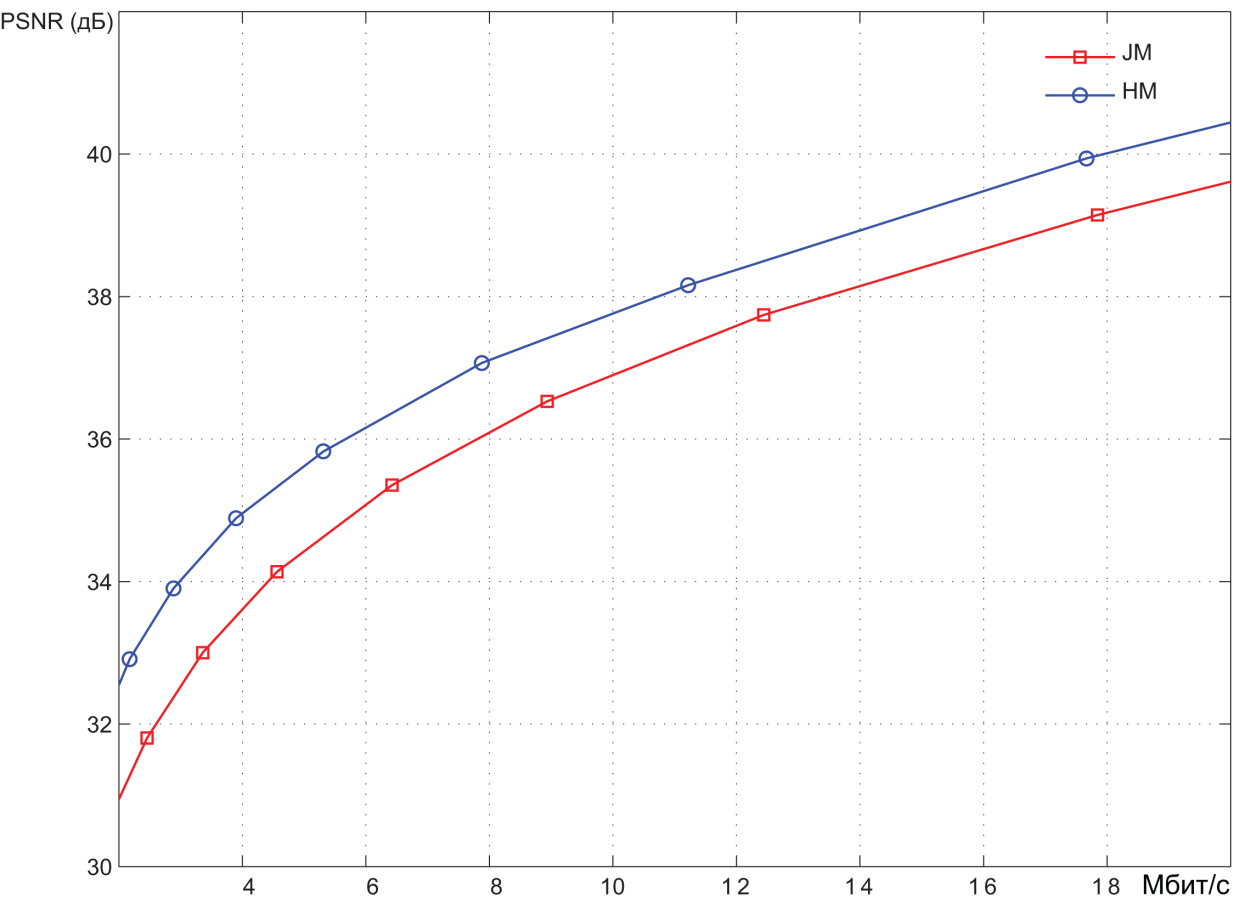Pic. 5. Encoding results of the video sequence Aspen (1920x1080 30 frames per second)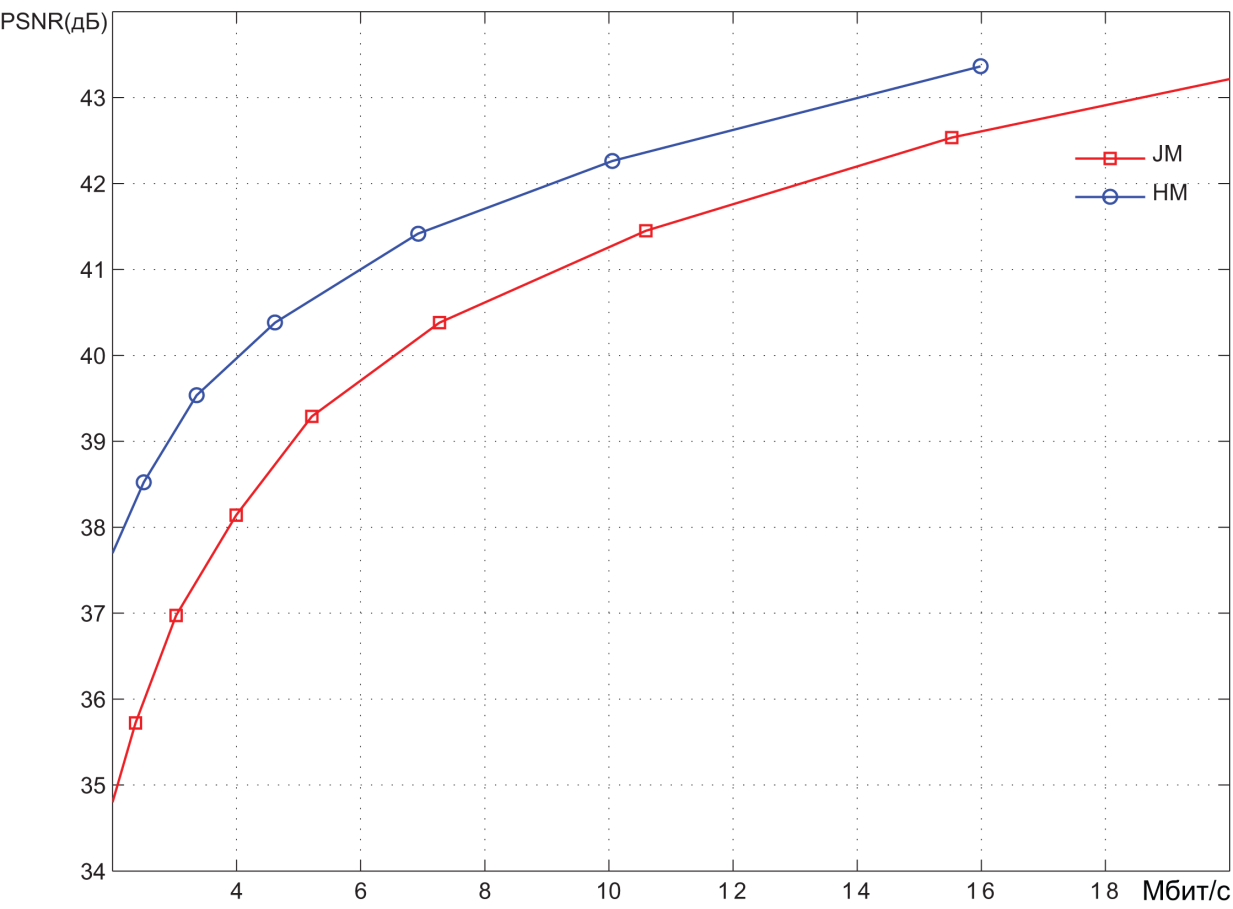Pic. 6. Encoding results of the video sequence BlueSky (1920x1080 25 frames per second)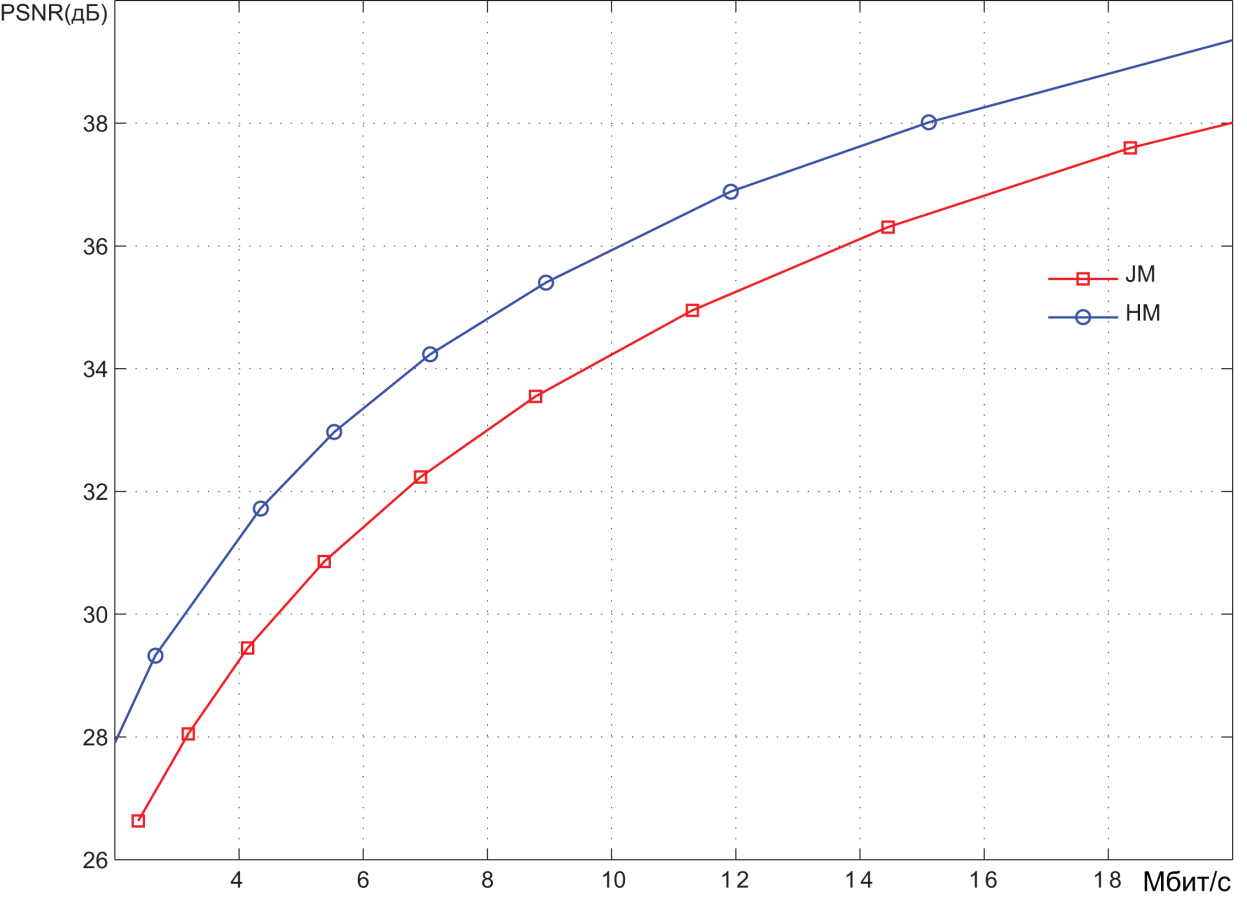Pic. 7. Encoding results of the video sequence PeopleOnStreet (1920x1080 30 frames per second)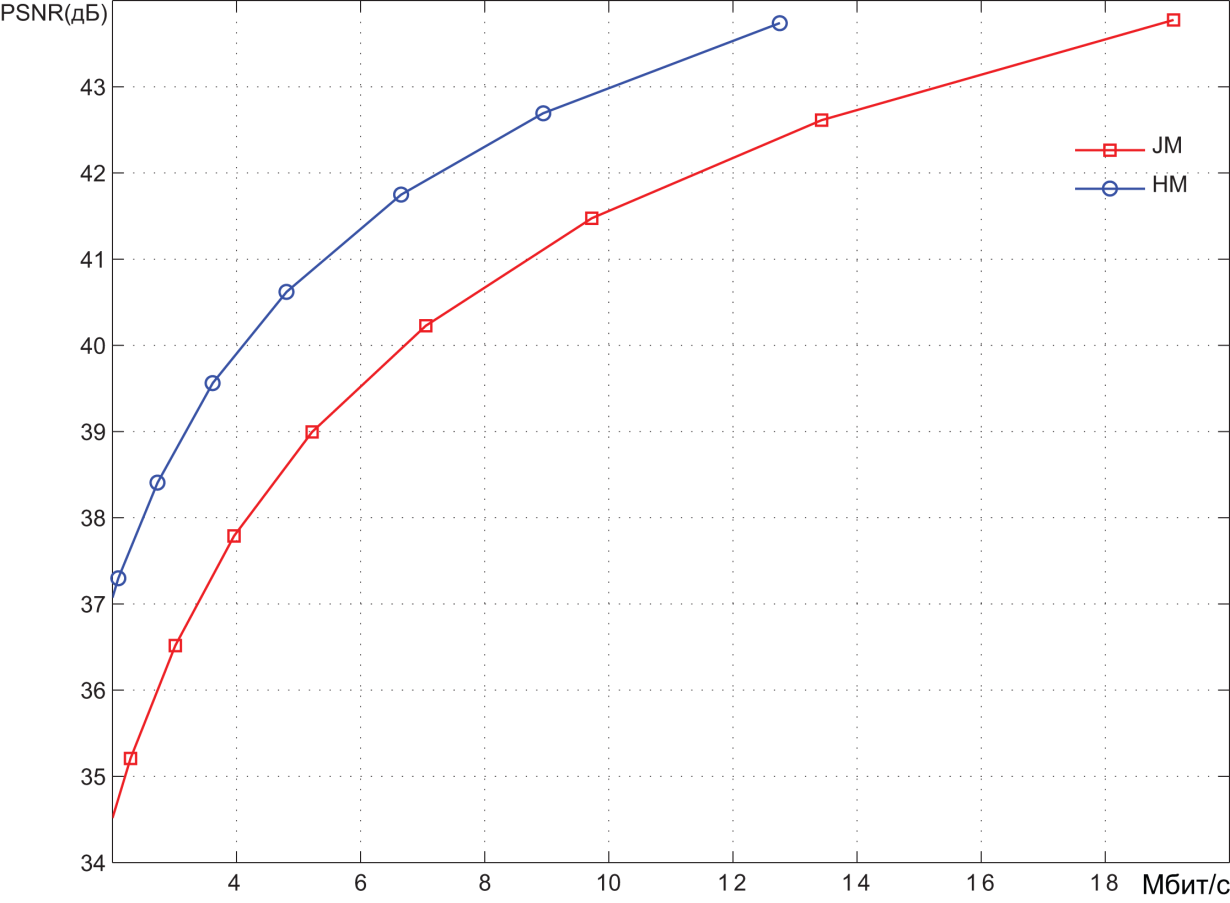Pic. 8. Encoding results of the video sequence Traffic (1920x1080 30 frames per second)

Coding was performed at different quantization values of spectral coefficients, hence with different levels of video image distortion. The results are presented in Bitrate(mbps) - PSNR(dB) coordinates. PSNR values characterize the degree of distortion.

On average, it can be stated that the PSNR range below 36 dB corresponds to a high level of distortion, i.e. low quality video images. The range of 36 to 40 dB corresponds to the average quality. With PSNR values above 40 dB, we can call it a high video quality.

We can roughly estimate the compression ratio provided by the encoding systems. In the medium quality area, the bit rate provided by the HEVC encoder is about 1.5 times less than the bit rate of the AVC encoder. Bitrate of an uncompressed video stream is easily determined as the product of the number of pixels in each video frame (1920 x 1080) by the number of bits required to represent each pixel (8 + 2 + 2 = 12), and the number of frames per second (30).

As a result, we get about 750 Mbps. It can be seen from the graphs that, in the area of average quality, the AVC encoder provides a bit rate of about 10-12 Mbit/s. Thus, the degree of video information compression is about 60-75 times. As already mentioned, the HEVC encoder provides compression ratio 1.5 times higher.

March 7, 2018

Chapter 2. Inter-frame prediction (Inter) in HEVC

Chapter 3. Spatial (Intra) prediction in HEVC

Chapter 4. Motion compensation in HEVC

Chapter 5. Post-processing in HEVC